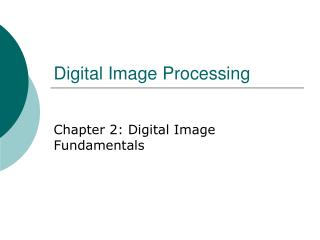DownloadDownload PresentationDigital Image Processing

# Digital Image Processing

Télécharger la présentation## Digital Image Processing

- - - - - - - - - - - - - - - - - - - - - - - - - - - E N D - - - - - - - - - - - - - - - - - - - - - - - - - - -
##### Presentation Transcript

1. Digital Image Processing Chapter 2: Digital Image Fundamentals

2. Elements of Visual Perception • Structure of the human eye

3. Rods and cones in the retina

4. Image formation in the eye

6. Brightness discrimination

7. Weber ratio

8. Perceived brightness

9. Simultaneous contrast

10. Optical illusion

11. Light and the Electromagnetic Spectrum

12. Wavelength

13. Image Sensing and Acquisition

14. Image acquisition using a single sensor

15. Using sensor strips

16. A simple image formation model

17. Illumination and reflectance • Illumination and transmissivity

18. Image Sampling and Quantization

19. Sampling and quantization

20. Representing digital images

21. Saturation and noise

22. Number of storage bits

23. Spatial and gray-level resolution

24. Subsampled and resampled

25. Reducing spatial resolution

26. Varying the number of gray levels

27. Varying the number of gray levels

28. N and k in different-details images

29. Isopreference

30. Interpolations

31. Zooming and shrinking

32. Some Basic Relationships Between Pixels • Neighbors of a pixel • : 4-neighbors of p , , , : four diagonal neighbors of p , , , : 8-neighbors of p and

33. Adjacency • : The set of gray-level values used to define adjacency • 4-adjacency: Two pixels p and q with values from V are 4-adjacency if q is in the set • 8-adjacency: Two pixels p and q with values from V are 8-adjacency if q is in the set

34. m-adjacency (mixed adjacency): Two pixels p and q with values from V are m-adjacency if • q is in , or • q is in and the set has no pixels whose values are from V

35. Subset adjacency • S1 and S2 are adjacent if some pixel in S1 is adjacent to some pixel in S2 • Path • A path from p with coordinates to pixel q with coordinates is a sequence of distinct pixels with coordinates • , ,…, where = , = , and pixels and are adjacent

36. Region • We call R a region of the image if R is a connected set • Boundary • The boundary of a region R is the set of pixels in the region that have one or more neighbors that are not in R • Edge • Pixels with derivative values that exceed a preset threshold

37. Distance measures • Euclidean distance • City-block distance • Chessboard distance

38. distance: The shortest m-path between the points

39. An Introduction to the Mathematical Tools Used in Digital Image Processing • Linear operation • H is said to be a linear operator if, for any two images f and g and any two scalars a and b,

41. Arithmetic operations • Subtraction

42. Digital subtraction angiography